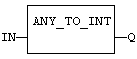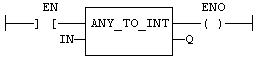# ANY_TO_INT / ANY_TO_UINT

Operator - Converts the input into 16 bit integer value.

## Inputs

IN : ANY  Input value

## Outputs

Q : INT  Value converted to 16 bit integer

## Remarks

For BOOL input data types, the output is 0 or 1. For REAL input data type, the output is the integer part of the input real. For TIME input data types, the result is the number of milliseconds. For STRING inputs, the output is the number represented by the string, or 0 if the string does not represent a valid number. In LD language, the conversion is executed only if the input rung (EN) is TRUE. The output rung (ENO) keeps the same value as the input rung. In IL Language, the ANY_TO_INT function converts the current result.

## ST Language

Q := ANY_TO_INT (IN);

## FBD Language## LD Language

(* The conversion is executed only if EN is TRUE *)
(* ENO keeps the same value as EN *)Op1: LD  IN
ANY_TO_INT
ST  Q